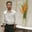Related Tags

html
css

# How to draw a circle using the SVG tag in HTMLBehzad Ahmad

## Overview

Scalable Vector Graphics (SVG) is a language that is used to describe 2D graphics and XML graphical applications, which the SVG viewer then renders. Many web browsers display SVG images just like images of .png, .jpg, and .gif formats.

The element <circle> is used to draw a circle with a given radius and a center point. A circle is drawn on the screen with the help of three parameters, namely the x and y coordinates of the center point and the radius of the circle.

### Syntax

<circle
cx="co-ordinates of x-axis"
cy="co-ordinates of y-axis"
</circle>



### Parameters

• cx: This defines the x-axis coordinate of the center of the circle. Its default value is 0.

• cy: This defines the y-axis coordinate of the center of the circle. Its default value is 0.

• radius: This defines the radius of the circle.

### Example

Let’s discuss a simple example to demonstrate the use of the element <circle>

### Explanation

The following is the explanation for the HTML of the code above:

• Line 4: We define the height and width of the SVG element.

• Line 5: We define the properties of the circle, namely the x and y coordinates of its center and the radius of the circle.

RELATED TAGS

html
css

CONTRIBUTORBehzad Ahmad
RELATED COURSES

View all Courses

Keep Exploring

Learn in-demand tech skills in half the time